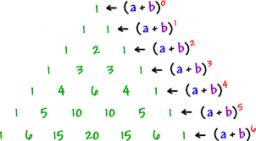# Subjects

Student makes a mathematical task for five minutes and the task from biology in twelve minutes. Another day he have homework in math and biology which consists of eleven tasks together. To do the job took hour and a half. How many tasks maked in each subject?Did you find an error or inaccuracy? Feel free to write us. Thank you!Tips to related online calculators
Do you have a system of equations and looking for calculator system of linear equations?
Do you solve Diofant problems and looking for a calculator of Diofant integer equations?